## Symbols

There are a lot of different symbols used in statistics. There could also be symbols you have used before in another class, but mean something different in stats. To clear some confusion, I compiled a list of the more common symbols used in intro CSU stat courses.

NameSymbolDescription
Population Mean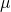A parameter, the population average.
Sample MeanA statistic, the sample average.
Population Standard Deviation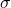A parameter, which measures the variability in the population.
Sample Standard Deviation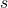A statistic, which measures the variability in the sample
Sample Size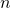The number of observations in an experiment.
Pooled Standard Deviation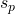A weighted average of two groups standard deviations.
T ValueCan represent a test statistic when performing a t-test or the critical value when creating a CI.
Z Value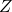Can represent a z-score or a test statistic.
Sample Correlation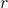Measures the linear relationship between two variables.
Population Correlation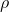Measures the linear relationship between two variables.
ProportionThe number of "successes" over the total observations.
Null Hypothesis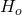The assumed hypothesis when performing a hypothesis test.
Alternative Hypothesis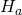The hypothesis we are trying to "disprove" in a hypothesis test.
Estimated SlopeThe estimated slope in the line of best fit.
Estimated Y-Intercept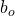The estimated y-intercept in the line of best fit.
Population Slope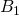The theoretical slope in a linear regression model.
Population Y-Intercept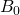The theoretical y-intercept in a linear regression model.
Coefficient of Determination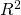The proportion of variability in the response that can be explained by the model.
Random ErrorThe random error term that is added in the theoretical linear regression model.
F ValueThe test statistic used when performing ANOVA.